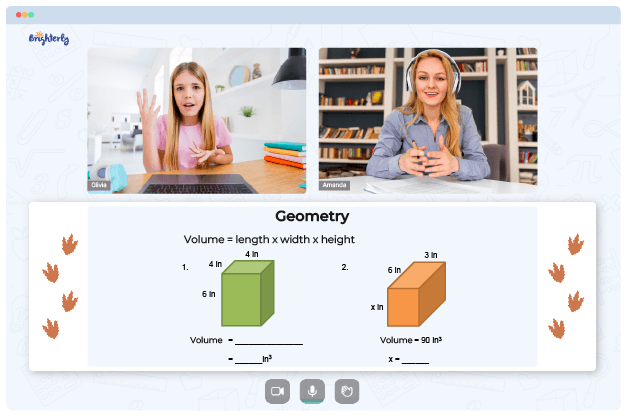# Area Of A Triangle Worksheets

A triangle is a geometric shape in which three straight segments meet. Their meeting points are also known as angles. And there are different kinds of triangles students learn in math class.

But without the right tools, the learning process can be long and tedious. An area of a triangle worksheet allows students to work with those triangles more easily and solve related problems.

## Finding the Area of a Triangle

A triangle’s area equals half the area of ​​a rectangle. Thus, the area of ​​a triangle is deduced from the formula A = ½ BH, where A represents the area, B represents the base, and H represents the triangle’s height. When parents and teachers teach children the concept of the area of ​​a triangle, it can be difficult for children to understand the idea. So, the introduction of an area of triangles worksheet helps children learn math concepts quickly.

Math for Kids

Is Your Child Struggling With Math?
1:1 Online Math Tutoring## The Benefits of Area of a Triangle Worksheets

A worksheet on triangles helps children easily understand geometry concepts and theories. Its depth will enable children to practice the method repeatedly, reinforcing vital math skills. The use of worksheets also helps students understand the technique and demonstrate that they can make a difference using the same process. These worksheets allow children to master the ideas effectively.

Children find it easy to learn the area of ​​a triangle when they use an area of triangle worksheet. With worksheets, kids can improvise to improve the knowledge of concepts they are learning. The worksheets are powerful tools to get kids involved in learning for longer, and kids can learn about their strengths and weaknesses as they practice the papers.### Area Of A Triangle Worksheets PDF

Area Of A Triangle Worksheet### Area Of A Triangle Worksheets PDF

Area Of Triangle Worksheet### Area Of A Triangle Worksheets PDF

Area Of Triangles Worksheet### Area Of A Triangle Worksheets PDF

Area Of Right Triangle Worksheet

The questions you can find in any area of right triangle worksheet can be simple or complex. Solving triangle-based questions helps students build solid knowledge of geometry concepts. Students improve their understanding as they learn to calculate the area of ​​a triangle.

### Area and Perimeter Worksheets

Need help with Geometry?• Does your child need extra help with understanding geometry lessons?
• Start studying with an online tutor.

Is your child finding it hard to grasp the concept of geometry? An online tutor could be of assistance.